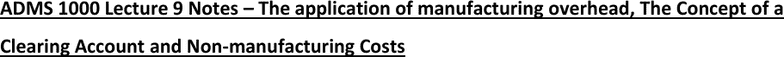Class Notes (1,100,000)
CA (640,000)
York (40,000)
Lecture 9

# ADMS 1000 Lecture Notes - Lecture 9: General Ledger, Subledger, Oil PlatformPremium

Department
Course Code
Professor
Keith Lehrer
Lecture
9

This preview shows page 1. to view the full 4 pages of the document.ADMS 1000 Lecture 9 Notes The application of manufacturing overhead, The Concept of a
Clearing Account and Non-manufacturing Costs
Introduction
control account rather than to Work in Process, how are manufacturing overhead costs
assigned to Work in Process?
Recall from our discussion earlier in the chapter that a predetermined overhead rate is
established at the beginning of each year.
The rate is calculated by dividing the estimated total manufacturing overhead cost for
the year by the estimated total units in the allocation base (measured in machine-hours,
direct labour-hours, or some other base).
The predetermined overhead rate is then used to apply overhead costs to jobs.
For example, if direct labour-hours are the allocation base, overhead cost is applied to
each job by multiplying the number of direct labour-hours charged to the job by the
To illustrate, assume that Rand Company has used machine-hours in computing its
predetermined overhead rate and that this rate is \$6 per machine-hour.
Also assume that during April, 10,000 machine-hours were worked on Job A and 5,000
machine-hours were worked on Job B (a total of 15,000 machine-hours).
Thus, \$90,000 in overhead cost (15,000 machine-hours × \$6 = \$90,000) would be
applied to Work in Process.
The flow of costs through the Manufacturing Overhead account is detailed in Exhibit 5
8.
The “actual overhead costs” in the Manufacturing Overhead account shown in Exhibit
58 are the costs that were added to the account in entries (2) through (6).